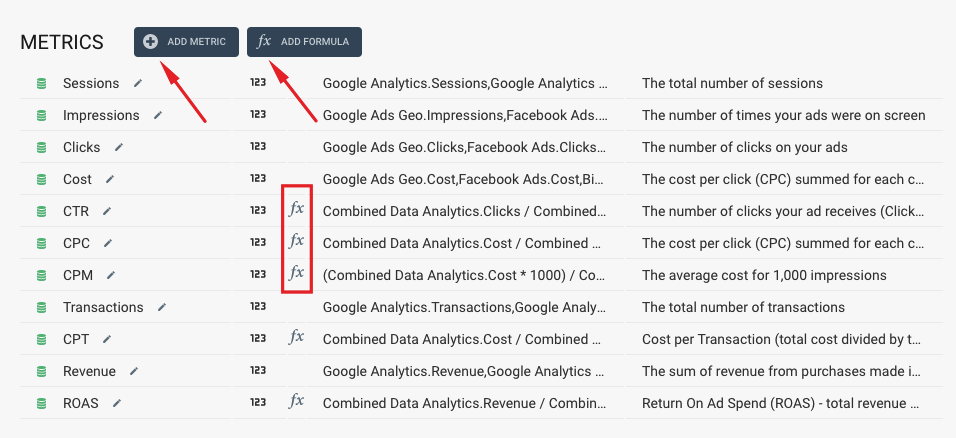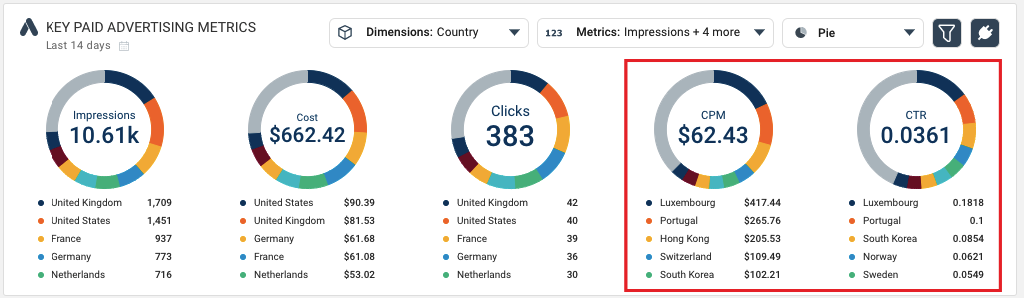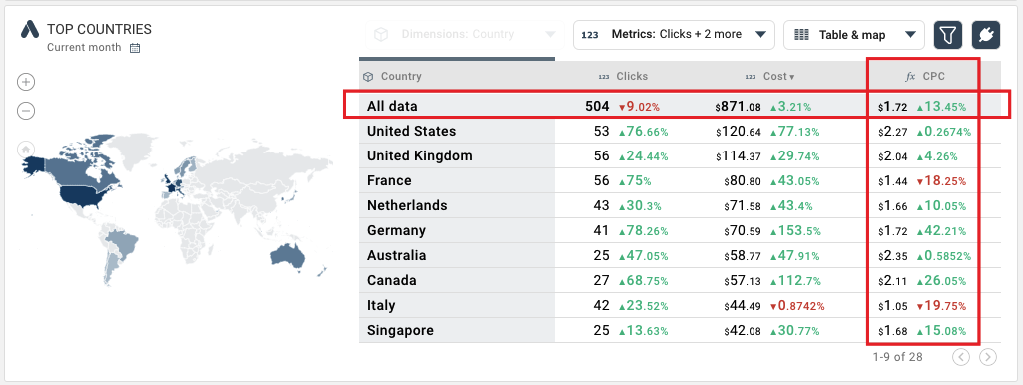EnglishTRIALTRIALEnglish

# METRIC TYPES: STANDARD METRICS VS FORMULAS

Knowledge BaseMetric Types: Standard Metrics Vs Formulas

## Using rules: Standard Metrics vs Formulas

There are two types of rules in the Octoboard PPC Analytics Module - Standard Metrics and Formulas. Formulas are marked with a formula icon and can be created using the ADD FORMULA button shown below:• Standard metrics are calculated once at the time of data retrieval from connected cloud applications.
• Formulas are recalculated every time data is displayed.

You should use Formulas if your new metric uses division or if you can not add or average values during data aggregation (you cannot calculate an accurate CostPerClick value for your European market by averaging CPC values of European countries). Formulas are recalculated every time before data is displayed in widgets or tables.

In the list of metrics above, CTR, CPC, CPM, CPT and ROAS are all formulas because they all involve data division (CPC = Cost / Clicks) and because if you would like to calculate CostPerClick for five countries, for example, you cannot add up or average CPC values to get the total CPC. The image below illustrates this example.Here CPC and CTR for 5 countries cannot be calculated during data collection. Instead, Octoboard will collect Cost, Clicks and Impressions (they are Standard Metrics) and then use Formulas to recalculate accurate CPM and CTR every time it displays aggregated values in tables or data widgets.The Data Explorer widget above shows aggregated CPC formula value (\$1.72). The CPC data field is not stored in the database. It is calculated when the Data Explorer is displayed. In our Data Warehouse database, there will be no CPC data field.

Octoboard does not export formula-based data fields to Google Data Studio, Looker or Google BigQuery. Instead, our PPC Data Analytics module exports formula definitions in the format that external Business Intelligence products would accept. BI systems would use our formula definitions to recalculate correct aggregated values before data is displayed.

For more details on PPC Data Analytics export, please read this tutorial.

There is one more case that requires the use of Formulas. If you would like to create a metric in the Combined Data Stream based on an already existing metric of the stream, you should create a Formula. Using a formula in Combined Data Stream is covered in this tutorial.SUGGESTED TUTORIALS